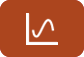## Error message

• Deprecated function: Methods with the same name as their class will not be constructors in a future version of PHP; ctools_context has a deprecated constructor in require_once() (line 127 of /home/tusa/public_html/sites/all/modules/ctools/ctools.module).
• Deprecated function: Methods with the same name as their class will not be constructors in a future version of PHP; ctools_context_required has a deprecated constructor in require_once() (line 127 of /home/tusa/public_html/sites/all/modules/ctools/ctools.module).
• Deprecated function: Methods with the same name as their class will not be constructors in a future version of PHP; ctools_context_optional has a deprecated constructor in require_once() (line 127 of /home/tusa/public_html/sites/all/modules/ctools/ctools.module).
• Deprecated function: Methods with the same name as their class will not be constructors in a future version of PHP; panels_cache_object has a deprecated constructor in require_once() (line 127 of /home/tusa/public_html/sites/all/modules/ctools/ctools.module).

# Free Trigonometry Worksheets100% Free Trigonometry Worksheets with Answer Keys

Looking for some free trigonometry resources? Tutor-USA offers 100% Free Trigonometry Worksheets for use by high school trigonometry teachers, students, and homeschool parents.

Current worksheets topics include logarithms, circular functions, exponential functions, and inverse functions. Additional worksheets will be added soon.

This free calculus worksheet contains problems on integration. Integrals in this worksheet result in answers that contain inverse trigonometry functions.
Worksheet (Calculus)
Problems: 12
This free trigonometry worksheets contains problems on applications of exponential functions. Students must find interest compounded annually, semi-annually, quarterly, and continuously. Other...
Worksheet (Trigonometry)
Problems: 10
In this free trigonometry worksheet, students must complete problems involving logarithmic functions. Students must express logarithmic functions as exponential functions, exponential functions as...
Worksheet (Trigonometry)
Problems: 15
This free trigonometry worksheet contains problems where students must find the exact values of inverse trigonometric functions. Problems in this worksheet use the notation sin<sup>-1</sup...
Worksheet (Trigonometry)
Problems: 20
<p> This free trigonometry worksheets features problems on inverse trigonometric functions. Functions use the notation sin<sup>-1</sup> instead of arcsin.&nbsp; Students must...
Worksheet (Trigonometry)
Problems: 23
This free trigonometry worksheet contains problems where students must find the value of inverse circular trigonometric functions. Students must apply the properties of arcsin, arccos, and arctan...
Worksheet (Trigonometry)